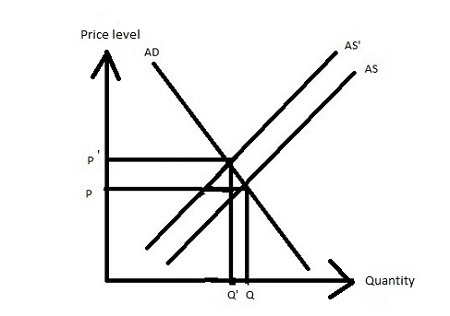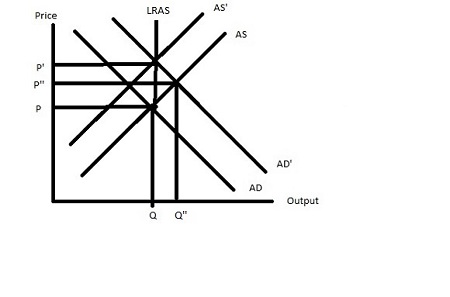# Rising oil prices will lead to decreased employment, lower wage rates and decreased real money...

## Question:

Rising oil prices will lead to decreased employment, lower wage rates and decreased real money balances.

Comment on this statement with the help of an AD-AS diagram and explain the short-run and long-run adjustment processes.

## Aggregate Demand and Aggregate Supply:

As the price level increases then the total quantity of all goods demanded decreases and as the price level decreases then the total quantity of all goods demanded increases shows that the aggregate demand curve is downward sloping and it has a negative slope.

As the price level increases then the total quantity of all goods supplied increases and as the price level decreases then total quantity of all good demanded decreases shows that the aggregate supply curve is upward sloping and it has a positive slope.

## Answer and Explanation:

In the short run, as the price of oil rises then the aggregate supply curve shifts leftward with the same aggregate demand curve such that the price increases and quantity decrease.In the long run, the long-run aggregate supply is vertical such that as the price of oil rises then the short-run aggregate supply shifts leftward and at the same time the aggregate demand curve shifts rightward which increase the price and quantity remains the same or equilibrium quantity is achieved.#### Learn more about this topic:Aggregate Supply and Aggregate Demand (AS-AD) Model

from Economics 102: Macroeconomics

Chapter 7 / Lesson 3
58K